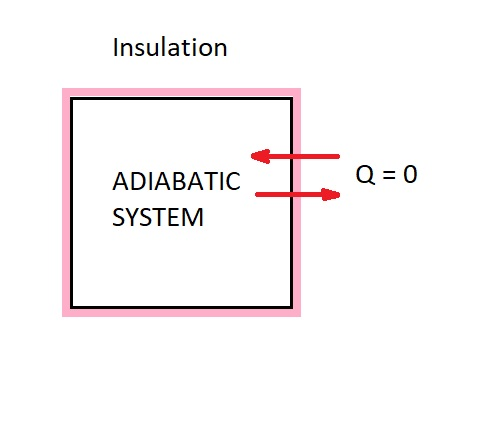# Heat Energy

There are two main forms of energy, work and heat.   When you are analyzing a system of processes it is important that you are able to tell the difference between the two. In this article I am going to discuss heat energy.

### What is Heat?

Now imagine that you just cooked dinner.  The food when it comes off the stove or out of the oven should be hot. However, let’s say you get distracted for about 20 minutes before you get the chance to eat it.  Once you get back to the food it won’t be hot anymore instead it will warm or possibly cold.  So what happened?  Simply the heat energy within the food was transferred to the surrounding environment.  This energy will continue to transfer until both your food and the surrounding environment are at the same temperature.  In other word, the food has reached equilibrium with the surrounding environment.   Finally, it is important to note that the transfer of heat energy always occurs from hot to cold.

From the above example the following definition for heat can be derived.  Heat is a form of energy that is transferred between two systems, or a system and it surroundings due to a temperature difference.  As a result, heat energy will not transfer if there is no temperature difference present.

An adiabatic process is a type of thermodynamic process.  For a process to be considered adiabatic heat transfer across the boundary must not occur.  In order for this process to occur one of the two conditions must be met.  The first condition is the system must well insulated so that only a negligible amount of heat passes over the systems boundary.  However, if this is not case than for an adiabatic process to occur the system and its surrounding must be at the same temperature.### Heat Energy Units

Due to the fact that heat is a form of energy its units will written as kJ for the SI system or Btu for the English system.  In addition, heat transfer can be written in a per unit mass for the system.

(Eq 1) $q=\frac{Q}{m}$ (kJ/kg)

$Q$ = Heat Energy

$m$ = mass

Finally, there are also cases where you will want to describe the rate of heat transfer per unit time.  When this is the case you will need to integrate the heat energy per unit time.

(Eq 2) $Q=\int{}^{t_2}_{t_1}~\dot{Q}dt$ (kJ)

or

(Eq 3) $Q=\dot{Q}Δt$ (kJ)

### Types of Heat Transfer

There are three types of heat transfer; conduction, convection, and radiation.  Conduction is the transfer of heat through a solid body.  On the other hand, convection is the transfer of heat to a moving fluid from a solid body.  Finally, radiation is the transfer of heat through the electromagnetic spectrum.  Radiation can occur in both a vacuum as well as through matter such as air or water.